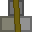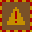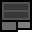# Account 396705

## BalanceMetal: 0s5 = 5Explosives: 55s15 = 895RC Turrets: 0

## Transfer

 PIN Receiver ReferenceMetal 16 × +Explosives 16 × +RC Turrets

## Create new account token

PIN:

New accounts cost 2 stacks (32 units) metal. You must give the token directly to the other player. Do not create the account yourself and give them the details. If you are found to do this, your account will be closed and all your items taken. You are responsible for ensuring the player you provide a new account token to knows how to use dredbank properly. If many players are found who don't know how to use dredbank properly, and they all got their accounts from one player, there may be a punishment against the player who provided their accounts.

## Transactions

Type Counterparty Account Reference NumberMetalExplosivesRC Turrets
Sent 803203 777 0s0 = 0 50s0 = 800 0
Sent 801425 777 33s0 = 528 0s0 = 0 0
Sent 321000 0 2s0 = 32 0s0 = 0 0
Sent 915654 111 95s0 = 1520 0s0 = 0 0
Sent 321000 0 2s0 = 32 0s0 = 0 0
Received 333000 123 26s0 = 416 0s0 = 0 0
Deposit 0 85s15 = 1375 0s0 = 0 0
Sent 333000 777 35s10 = 570 4s7 = 71 0
Received 917583 0 9s0 = 144 0s0 = 0 0
Received 917583 0 0s0 = 0 2s0 = 32 0
Received 917583 0 47s0 = 752 0s0 = 0 0
Deposit 0 0s0 = 0 63s15 = 1023 0
Received 483744 1000 0s0 = 0 15s0 = 240 0
Received 483744 1000 0s0 = 0 10s0 = 160 0
Sent 483744 1111 79s12 = 1276 30s6 = 486 0
Deposit 0 10s0 = 160 0s0 = 0 0
Deposit 0 0s0 = 0 48s13 = 781 0
Deposit 0 0s0 = 0 1s0 = 16 0
Deposit 0 69s12 = 1116 0s0 = 0 0